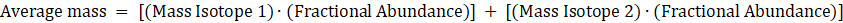# Problem: The atomic mass of fluorine is 18.998 amu and its mass spectrum shows a large peak at this mass. The atomic mass of chlorine is 35.45 amu, yet the mass spectrum of chlorine does not show a peak at this mass.Explain the difference.

###### FREE Expert Solution

Mass spectroscopy is an analytical technique that plots ion signal (relative abundance) as a function of mass-to-charge ratio.

To understand why fluorine has a large peak at its atomic mass 18.998 amu while chlorine does not have a large peak at 35.45 amu, we will determine solve for each atom’s average mass using their relative abundance. The formula for average mass is:94% (191 ratings)###### Problem Details

The atomic mass of fluorine is 18.998 amu and its mass spectrum shows a large peak at this mass. The atomic mass of chlorine is 35.45 amu, yet the mass spectrum of chlorine does not show a peak at this mass.

Explain the difference.